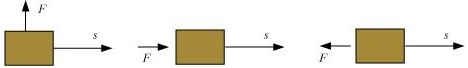Worksheet: Work and Energy

# Worksheet: Work and Energy - Science Class 9

Q.1. Fill in the blank
(i) When a ball is thrown upwards, ________ energy is transformed into ________ energy.
(ii) The sum of the potential and kinetic energies of a body is called ________ energy.
(iii) Work is measured as a product of ________ and _________.
(iv) The electricity meter installed in our home measures electric energy in the units of ______.
(v) The work done on a body moving in a circular path is ___________.

Q.2. How are Joule (J) and ergs (erg) related?
(a) 1J = 107erg
(b) 1erg = 107J
(c) 1J = 10-7erg
(d) None

Q.3.  If the force is applied at an angle θ then work done is
(a) W = FS Cos θ  F = Force
(b) W = FS θ S =  Distance
(c) W = FS Sin  θ W = work
(d) None

Q.4. If a body is stored at a height ‘h’ then it will posses:
(a) Kinetic energy
(b) Potential energy
(c) Both
(d) None

Q.5. If the body starts from rest, then change in its kinetic energy is
(a) Positive
(b) Negative
(c) Zero
(d) May be Positive or negative depending upon the mass of the body

Q.6. When do we say that work is done?

Q.7. Define 1 J of work.

Q.8. What is the kinetic energy of an object?

Q.9. What is power?

Q.10. An object thrown at a certain angle to the ground moves in a curved path and falls back to the ground. The initial and the final points of the path of the object lie on the same horizontal line. What is the work done by the force of gravity on the object?

Q.11. Illustrate the law of conservation of energy by discussing the energy changes which occur when we draw a pendulum bob to one side and allow it to oscillate. Why does the bob eventually come to rest? What happens to its energy eventually? Is it a violation of the law of conservation of energy?

Q.12. Define average power.

Q.13.  In each of the following a force, F is acting on an object of mass, m. The direction of displacement is from west to east shown by the longer arrow. Observe the diagrams carefully and state whether the work done by the force is negative, positive or zero.Q.14. Define 1 watt of power.

Q.15. A battery lights a bulb. Describe the energy changes involved in the process.

The document Worksheet: Work and Energy | Science Class 9 is a part of the Class 9 Course Science Class 9.
All you need of Class 9 at this link: Class 9

## Science Class 9

66 videos|352 docs|97 tests

## FAQs on Worksheet: Work and Energy - Science Class 9

 1. What is work and energy?Ans. Work is defined as the transfer of energy that occurs when a force is applied to an object and it causes the object to move in the direction of the force. Energy, on the other hand, is the ability to do work or cause change. It can exist in various forms such as kinetic energy, potential energy, thermal energy, etc.
 2. What is the relationship between work and energy?Ans. The relationship between work and energy is that work done on an object transfers energy to the object. When work is done on an object, it increases the object's energy. Similarly, when work is done by an object, it decreases the object's energy. The work-energy theorem states that the work done on an object is equal to the change in its kinetic energy.
 3. How is work calculated?Ans. Work is calculated by multiplying the force applied to an object by the distance the object moves in the direction of the force. The formula for work is: work = force × distance × cosθ, where θ is the angle between the force and the direction of motion.
 4. What are the different forms of energy?Ans. There are several different forms of energy, including: - Kinetic energy: the energy of an object in motion. - Potential energy: the energy that an object possesses due to its position or state. - Thermal energy: the energy associated with the motion of particles in a substance. - Chemical energy: the energy stored in the bonds of chemical compounds. - Electrical energy: the energy associated with the flow of electric charge. - Nuclear energy: the energy released during nuclear reactions.
 5. How is energy conserved in a closed system?Ans. In a closed system, energy is conserved, which means it cannot be created or destroyed, only transferred or converted from one form to another. This is known as the law of conservation of energy. For example, if a ball is dropped from a certain height, the potential energy it has at the top is converted into kinetic energy as it falls. At the bottom, the kinetic energy is at its maximum while the potential energy is zero, but the total energy remains constant.

## Science Class 9

66 videos|352 docs|97 testsExplore Courses for Class 9 examSignup to see your scores go up within 7 days! Learn & Practice with 1000+ FREE Notes, Videos & Tests.
10M+ students study on EduRev
Track your progress, build streaks, highlight & save important lessons and more!
Related Searches

,

,

,

,

,

,

,

,

,

,

,

,

,

,

,

,

,

,

,

,

,

;